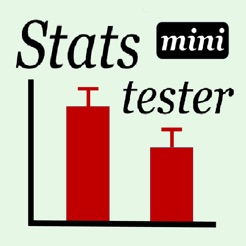## iPhone Screenshots

•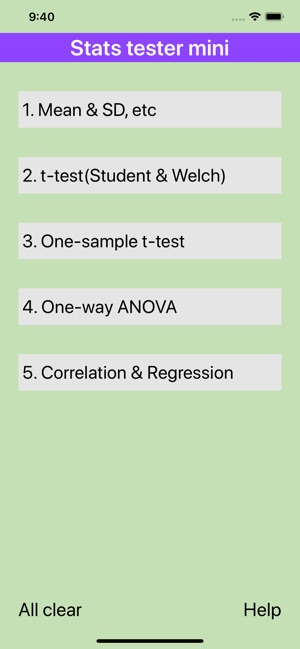•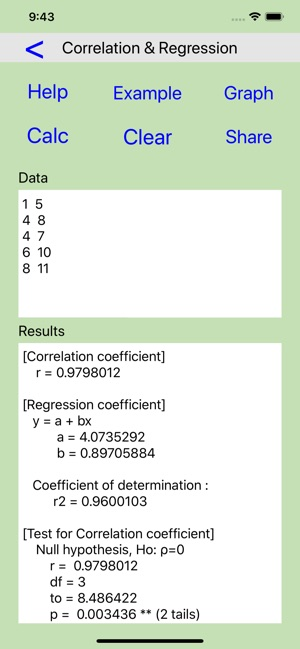•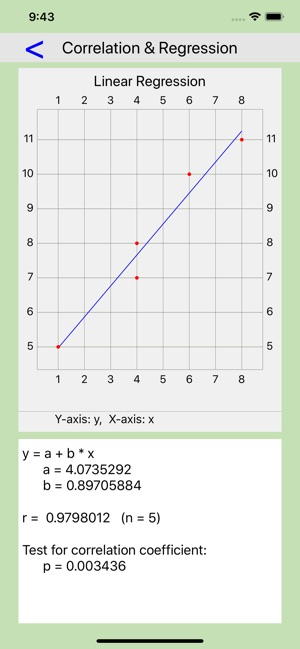••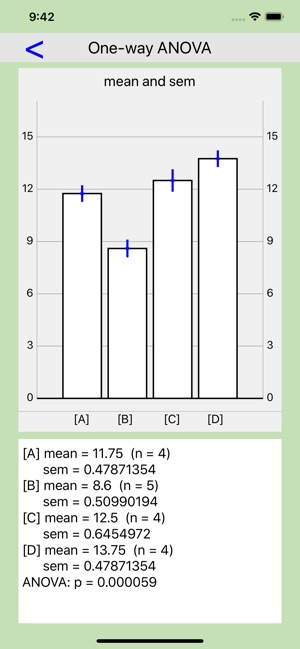•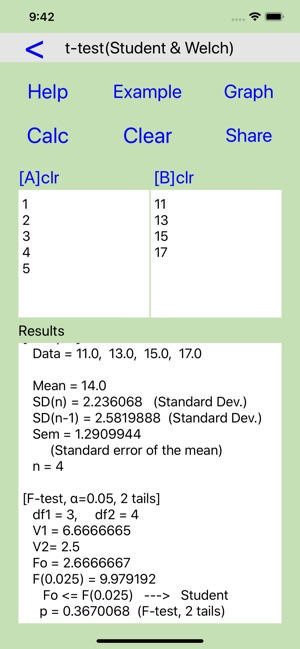•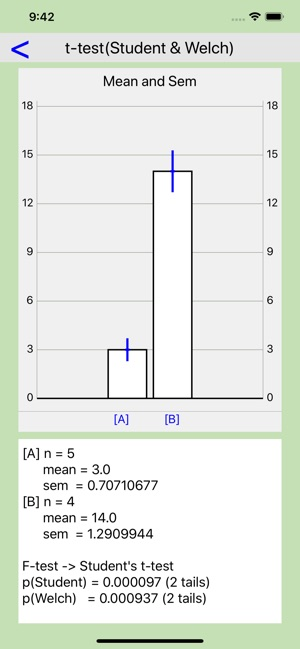## Description

By using Stats tester mini, you can perform statistics tests and obtain graphs by an easy operation. This app has the following features.

1. Each statistics method has useful [Help], [Example], [Share] and [Graph] buttons.

2. As one input window accepts one group data (max = 100), it is easy to edit the entered data (addition, deletion or modification).

3. Entered data and results calculated by each method are memorized until the next use. This function is a feature of this app and it is very convenient.

Statistic methods

1. Mean & SD, etc
1) Arithmetic, Geometric and Harmonic means
2) Standard Deviation (2)
3) Standard Error of the mean (sem)
4) 95 and 99% Confidence interval (CI)
5) Sum
6) Sum of Square
7) Variance, Unbiased variance
8) Coefficient of variation (%CV)
8) Skewness (2)
9) Kurtosis (2)

2. t-test (Student & Welch)
1) Mean
2) Standard deviation (2)
3) Standard error of the mean (sem)
4) F-test to compare 2 variances --> p value
5) Student t-test --> p value
6) Welch's procedure --> p value

3. One-sample t-test
1) Mean
2) Standard deviation (2)
3) Standard error of the mean (sem)
4) One-sample t-test --> p value
5) Confidence interval (CI)

4. One-way ANOVA (Analysis of variance)
1) Mean
2) Standard error of the mean (sem)
3) Analysis of variance --> p value
4) ANOVA table

5. Correlation & Regression
1) Correlation coefficient
2) Regression coefficient
3) Coefficient of determination
4) Test for correlation coefficient --> p value

## Information

Seller
Hideki Ono
Size
14 MB
Category
Education
Compatibility

Requires iOS 11.0 or later. Compatible with iPhone, iPad, and iPod touch.

Languages

English

Age Rating
Rated 4+
Copyright
© 2019 Hideki Ono (BMP group)
Price
Free

## Supports

•